4 篇文章 0 订阅

c语言实现推箱子小游戏

先让大家看一下效果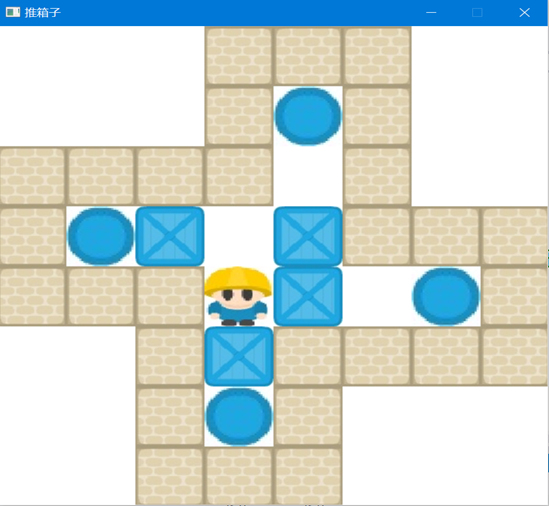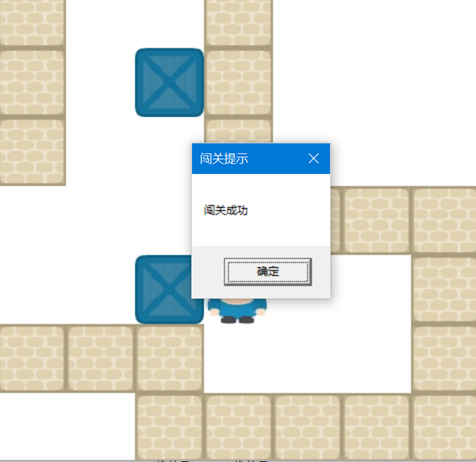下面是实现推箱子小游戏的全部代码

关于本游戏的resource.h ，这个是需要我们导入图片后，才会有的头文件，下面是关于本游戏的图片素材，我会演示一下VS 如何导入图片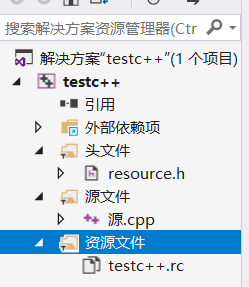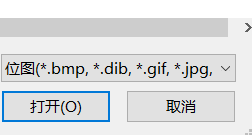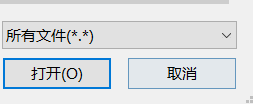2程序说明

2.1 按钮设计

(1)上：通过按w或W或 来移动小人往上移动。
(1)下：通过按s或S或 来移动小人往下移动。
(1)左：通过按a或A或 来移动小人往左移动。
(1)右：通过按d或D或 来移动小人往右移动。

2.2地图的绘制形成—初始化模块

(1)二维数组数字的意义
地图是以二维数组的形式进行存储的，不同的数字有着不同的含义。我将0~9解读为不同的图形。
0代表地图内的空位；
1代表墙壁；
3’代表目的地；
4代表箱子；
5代表小人；
7代表箱子在目的地；
8代表人在目的地。

2.3游戏操作模块

2.4游戏判断模块

#include <stdio.h>
#include <conio.h> //控制台输入输出的头文件
#include <graphics.h> //头文件图形库
#include "resource.h"
int Map = {
0, 0, 0, 1, 1, 1, 0, 0,
0, 0, 0, 1, 3, 1, 0, 0,
1, 1, 1, 1, 0, 1, 0, 0,
1, 3, 4, 0, 4, 1, 1, 1,
1, 1, 1, 5, 4, 0, 3, 1,
0, 0, 1, 4, 1, 1, 1, 1,
0, 0, 1, 3, 1, 0, 0, 0,
0, 0, 1, 1, 1, 0, 0, 0
};
void GameInitMap();//加载图片
void GamePaint(); //贴图
void GamePlay(); //操作
int GameJudgement(); //判断游戏是否结束
IMAGE BackImage, Wall;  //背景  墙
IMAGE Box, dBox; //箱子 箱子推到目的地
IMAGE Point; //目的地
IMAGE Person; //人
void GameInitMap(int live) //给出关卡数 用来判断是第几关
{
FILE *fp = fopen("Map.txt", "r");
if (NULL == fp)  //如果打开失败  异常处理
{
MessageBox(NULL, L"地图加载失败", NULL, NULL);
exit(0);
}
int FileOffset; // 文件的偏移
FileOffset = 66 * (live - 1);
fseek(fp, FileOffset, SEEK_SET);
int i, j;
char c;
for (i = 0; i < 8; i++)
{
for (j = 0; j < 8; j++)
{
c = fgetc(fp);
Map[i][j] = c - '0';
}
}
fclose(fp); //关闭文件
}
void GamePaint()
{
for (int i = 0; i < 8; ++i)
{
for (int j = 0; j < 8; ++j)
{
switch (Map[j][i])
{
case 0: //空地
break;
case 1: //墙壁
putimage(69 * i, 69 * j, &Wall);
break;
case 3://目的地
putimage(69 * i, 69 * j, &Point);
break;
case 4://箱子
putimage(69 * i, 69 * j, &Box);
break;
case 5://人物
putimage(69 * i, 69 * j, &Person);
break;
case 7://箱子在目的地上
putimage(69 * i, 69 * j, &dBox);
break;
case 8://人物在目的地上
putimage(69 * i, 69 * j, &Person);
break;
}
}
}
}
/*
0       空地
1       墙壁
3       目的地
4       箱子
5       人物
7       箱子在目的地
8       人在目的
*/
int GameJudgement()
{
for (int i = 0; i < 8; i++)
{
for (int j = 0; j < 8; j++)
{
if (Map[i][j] == 4) //判断 有没有落在空地上的箱子
{
return 1;  //游戏还没有结束
}
}
}
return 0;
}

void GamePlay()
{
char ch; //用来获取键盘输入的信息
int x, y; //描述当前坐标
int live = 1; //描述关卡
GameInitMap(live); //贴图
while (true)
{
BeginBatchDraw();
cleardevice();
putimage(0, 0, &BackImage);
if (!GameJudgement()) //判断 每一关是否玩完了
{
live++;  // 进入下一关
if (4 == live) //
{
GamePaint();// 绘图
FlushBatchDraw(); //批量绘图 防闪屏
MessageBox(GetHWnd(), L"闯关成功\r\n", L"闯关提示", MB_OK);
// 弹出对话框
FlushBatchDraw();
Sleep(5000);
closegraph(); //关闭窗口
exit(0); //结束程序
}
GameInitMap(live);
}
//批量绘图结束
GamePaint();
EndBatchDraw();
for (x = 0; x < 8; x++)
{
for (y = 0; y < 8; y++)
{	//如果人在目的地 或者 人在空地
if (Map[x][y] == 5 || Map[x][y] == 8)
{
break;
}
}
if (Map[x][y] == 5 || Map[x][y] == 8)
{
break;
}
}
ch = getch(); //获取键盘消息
//人在 Map[x][y]
switch (ch)
{
case 'w':
case 'W':
case 72://向上
if (Map[x - 1][y] == 0 || Map[x - 1][y] == 3)
{
Map[x][y] -= 5;
Map[x - 1][y] += 5;
}
else if (Map[x - 1][y] == 4 || Map[x - 1][y] == 7)
{
if (Map[x - 2][y] == 0 || Map[x - 2][y] == 3)
{
Map[x - 2][y] += 4;
Map[x - 1][y] += 1;
Map[x][y] -= 5;
}
}
break;
case 'a':
case 'A':
case 75://向左
if (Map[x][y - 1] == 0 || Map[x][y - 1] == 3)
{
Map[x][y] -= 5;
Map[x][y - 1] += 5;
}
else if (Map[x][y - 1] == 4 || Map[x][y - 1] == 7)
{
if (Map[x][y - 2] == 0 || Map[x][y - 2] == 3)
{
Map[x][y - 2] += 4;
Map[x][y - 1] += 1;
Map[x][y] -= 5;
}
}
break;
case 's':
case 'S':
case 80:  // 往下
if (Map[x + 1][y] == 0 || Map[x + 1][y] == 3)
{
Map[x][y] -= 5;
Map[x + 1][y] += 5;
}
else if (Map[x + 1][y] == 4 || Map[x + 1][y] == 7)
{
if (Map[x + 2][y] == 0 || Map[x + 2][y] == 3)
{
Map[x + 2][y] += 4;
Map[x + 1][y] += 1;
Map[x][y] -= 5;
}
}
break;
case 'd':
case 'D':
case 77:  //往右
if (Map[x][y + 1] == 0 || Map[x][y + 1] == 3)
{
Map[x][y] -= 5;
Map[x][y + 1] += 5;
}
else if (Map[x][y + 1] == 4 || Map[x][y + 1] == 7)
{
if (Map[x][y + 2] == 0 || Map[x][y + 2] == 3)
{
Map[x][y + 2] += 4;
Map[x][y + 1] += 1;
Map[x][y] -= 5;
}
}
break;
}
}
}
int main()
{
initgraph(550, 550);
GamePlay();
return 0;
}

0001110000013100111101001340411111154031001411110013100000111000
0111111101000001010343011104540110034301100000011111111100000000
0111100001001000010310001145100010401111100300011111000100011111
10-18
11-056905-20433
12-27150
06-174235
09-073万+
09-2911万+
04-17
05-106833
11-02
05-17301
12-177465
05-17259
02-052万+
01-17
05-25976
05-141451
07-26308
08-171万+Sundm@lhq

¥2 ¥4 ¥6 ¥10 ¥20余额支付 (余额：-- )扫码支付获取中扫码支付点击重新获取扫码支付1.余额是钱包充值的虚拟货币，按照1:1的比例进行支付金额的抵扣。
2.余额无法直接购买下载，可以购买VIP、C币套餐、付费专栏及课程。余额充值